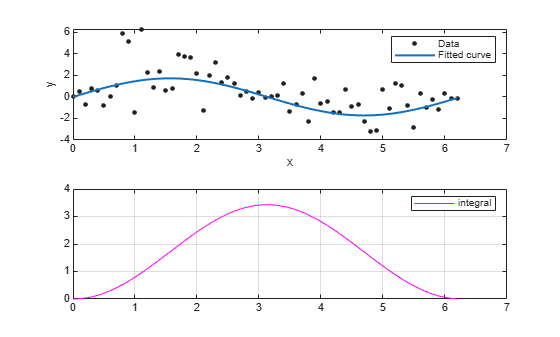integrate

Integrate cfit object

Description

example

int = integrate(fun,x,x0) integrates the cfit object fun at the points specified by the vector x, starting from x0, and returns the result in int.

Examples

collapse all

Create a baseline sinusoidal signal.

xdata = (0:.1:2*pi)';
y0 = sin(xdata);

Add response-dependent Gaussian noise to the signal.

noise = 2*y0.*randn(size(y0));
ydata = y0 + noise;

Fit the noisy data with a custom sinusoidal model.

f = fittype('a*sin(b*x)');
fit1 = fit(xdata,ydata,f,'StartPoint',[1 1]);

Find the integral of the fit at the predictors.

int = integrate(fit1,xdata,0);

Plot the data, the fit, and the integral.

subplot(2,1,1)
plot(fit1,xdata,ydata) % cfit plot method
subplot(2,1,2)
plot(xdata,int,'m') % double plot method
grid on
legend('integral')You can also compute integrals and plot them directly with the cfit plot method:

figure
plot(fit1,xdata,ydata,{'fit','integral'})The plot method, however, does not return data on the integral.

Input Arguments

collapse all

Function to integrate, specified as a cfit object.

Points at which to integrate the function, specified as a vector.

First point of integration, specified as a scalar.

Output Arguments

collapse all

Result of the integration, returned as a vector of the same size of x.